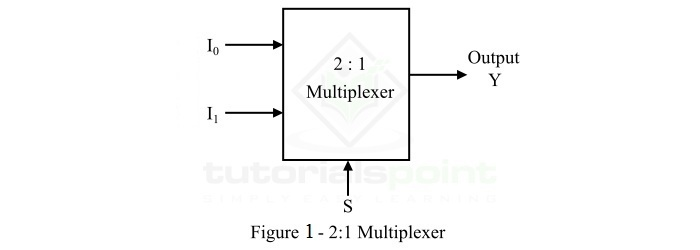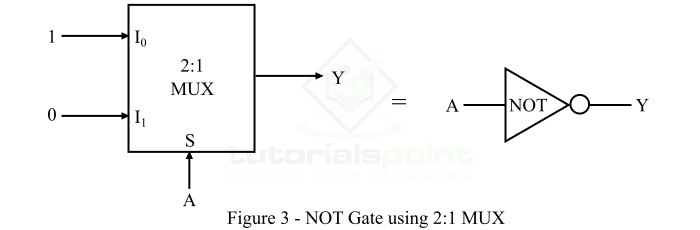# Realizing a NOT Gate Using a 2:1 MUX

Let' start this article with some basics of NOT gates and multiplexers.

## What is a Multiplexer?

In digital electronics, a multiplexer or MUX is a combinational logic circuit which accepts several data inputs and allows only one of them at a time to flow through the output line. Multiplexer (MUX) is also known as data selector because it selects one from many.

A multiplexer consists of 2n data input lines, n select lines, and 1 output line. Since, it converts 2n input lines into 1 output line. Therefore, it is also called many-to-one device.

Depending upon the number of input lines, there are several types of multiplexer present such as 2:1 MUX, 4:1 MUX, 8:1 MUX, and so on.

## 2:1 Multiplexer (MUX)

The logic block diagram of a 2:1 multiplexer is shown in Figure-1.A 2:1 MUX consists of 2 (21) data input lines designated by I0 and I1, 1 select line designated by S and 1 output line Y. The logic level either 0 or 1 applied to the select line S determines which input data will pass through the output line of the multiplexer.

The operation of the 2:1 MUX can be analyzed with the help of its truth table shown below.

Select Line (S) Output (Y)
0 I0
1 I1

From this truth table, we can conclude that,

• If select line S is connected to logic level 0, the data input connected to I0 will pass through the output line Y.

• If select line S is connected to logic level 1, the data input connected to I1 will pass through the output line Y.

Now, let us discuss about the NOT gate.

## What is a NOT Gate?

The NOT Gate is a basic logic gate that used in digital electronic circuits. The NOT gate has a single input and a single output. The output of the NOT gate is the logical inversion of its input. Hence, it is also called an inverter.

The standard logic symbol of the NOT gate has a triangle pointing to the right with a circle at its right end as shown in Figure-2. The circle at right corner is referred to as an inversion bubble.The NOT gate produces an output which is the complement or inversion of its input. For example, if we give a logic 0 at its input, it will provide a logic 1 at output. Similarly, when we give a logic 1 at input, it produces a logic 0 at output.

The operation of the NOT gate for all possible inputs can be analyzed with the help of its truth table which is given below.

Input (A) Output (Y = A')
0 1
1 0

From this truth table, we may derive the output equation of the NOT gate, i.e.

$$Y=\bar{A}=A'$$

Hence, it is clear that the NOT gate produces an output which is opposite of the applied input.

Now, we can discuss the realization of a NOT gate using a 2:1 MUX as we have enough knowledge of a 2:1 multiplexer and NOT gate required to do this.

## Realizing a NOT Gate by using a 2:1 MUX

The functional block diagram of a 2:1 multiplexer equivalent to the NOT gate is shown in Figure-3.There are two possible inputs, i.e. 0 and 1. We have applied 1 to the input line I0 and 0 to the input line I1. The input variable of the NOT gate is applied to the select line S of the MUX.

The operation of the 2:1 MUX as the NOT gate can be described as follows −

• When the input to the select line is A = 0, then the multiplexer will transmit the 1 applied at the input line I0 to the output line.

• When the input to the select line is A = 1, then the multiplexer will transmit the 0 applied at the input line I1 to the output line.

The truth table of the 2:1 MUX working as a NOT gate is given as follows,

S = A Output (Y)
0 1
1 0

Hence, the output expression of the NOT gate using 2:1 MUX will be,

$$Y=\bar{A}\cdot 1+A\cdot 0=\bar{A}$$

In this way, we can implement the NOT gate operation by using a 2:1 multiplexer.

Updated on: 19-Apr-2023

1K+ Views